## Essential Discriminant Divisors

Definition 8.1.5   A prime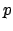is an if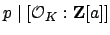for every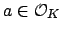.

Since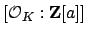is the absolute value of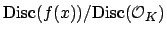, where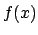is the characteristic polynomial of, an essential discriminant divisor divides the discriminant of the characteristic polynomial of any element of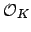.

Example 8.1.6 (Dedekind)   Let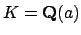be the cubic field defined by a root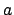of the polynomial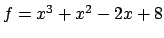. We will use , which implements the algorithm described in the previous section, to show that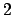is an essential discriminant divisor for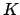.
   > K<a> := NumberField(x^3 + x^2 - 2*x + 8);
> OK := MaximalOrder(K);
> Factorization(2*OK);
[
<Prime Ideal of OK
Basis:
[2 0 0]
[0 1 0]
[0 0 1], 1>,
<Prime Ideal of OK
Basis:
[1 0 1]
[0 1 0]
[0 0 2], 1>,
<Prime Ideal of OK
Basis:
[1 0 1]
[0 1 1]
[0 0 2], 1>
]

Thus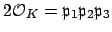, with the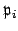distinct. Moreover, one can check that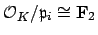. If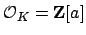for somewith minimal polynomial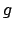, then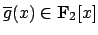must be a product of three distinct linear factors, which is impossible.

William Stein 2004-05-06